Abstracts

A quantum version of the Monty Hall problem, based upon the Positive Operator Valued Measures (POVM) formalism, is proposed. It is shown that basic normalization and symmetry arguments lead univocally to the associated POVM elements, and that the classical probabilities associated with the Monty Hall scenario are recovered for a natural choice of the measurement operators.

Quantum Measurements; Monty Hall Problem; Quantum Information Theory

Uma visão quântica do problema Monty Hall é proposta baseada no formalismo das Medidas Avaliadas do Operador Positivo (POVM). Demonstra-se que os argumentos de normalização básica e simetria levam de maneira inequívoca para elementos associados a POVM e que as probabilidades clássicas associadas ao cenário Monty Hall são recuperadas para uma escolha natural de medidas operadoras.

Medidas quânticas; Problema Monty Hall; Teoria quântica da informação

PHYSICAL SCIENCES

Positive operator valued measures and the quantum Monty Hall problem

Claudia ZanderI; Montserrat CasasII; Angel PlastinoIII; Angel R. PlastinoI

IDepartment of Physics, University of Pretoria, Pretoria 0002, South Africa

IIDepartament de Física and IMEDEA-CSIC, Universitat de les Illes Balears, 07122 Palma de Mallorca, Spain

IIIFacultad de Ciencias Exactas, Universidad Nacional de La Plata and Instituto de Física La Plata, Consejo Nacional de Investigaciones Científicas (CONICET) C.C. 727, 1900 La Plata, Argentina

ABSTRACT

A quantum version of the Monty Hall problem, based upon the Positive Operator Valued Measures (POVM) formalism, is proposed. It is shown that basic normalization and symmetry arguments lead univocally to the associated POVM elements, and that the classical probabilities associated with the Monty Hall scenario are recovered for a natural choice of the measurement operators.

Key words: Quantum Measurements, Monty Hall Problem, Quantum Information Theory.

RESUMO

Uma visão quântica do problema Monty Hall é proposta baseada no formalismo das Medidas Avaliadas do Operador Positivo (POVM). Demonstra-se que os argumentos de normalização básica e simetria levam de maneira inequívoca para elementos associados a POVM e que as probabilidades clássicas associadas ao cenário Monty Hall são recuperadas para uma escolha natural de medidas operadoras.

Palavras-chave: Medidas quânticas, Problema Monty Hall, Teoria quântica da informação.

INTRODUCTION

The physics of information has been the focus of intensive research in recent years (Frieden 2004, Leff and Rex 2003, Nielsen and Chuang 2000, Plastino and Daffertshofer 2004, von Baeyer 2003). The discovery of novel and counter-intuitive ways of processing and transmitting (quantum) information (Nielsen and Chuang 2000) has greatly increased the interest in this emerging field. Probabilistic concepts play, of course, a prominent role in this multidisciplinary area of science. The quantum mechanical formalism itself can be regarded as a generalization of classical probability theory. From this point of view, classical probabilistic settings can be "quantized" (Meyer 1999). The extension of classical probability problems to quantum mechanical scenarios (and the study of classical analogues of quantum mechanical information processes) illuminates fundamental issues concerning the relation between classical and quantum probabilities (Meyer 1999, Plastino and Daffertshofer 2004). Classical probability puzzles have also been used in order to clarify the application to statistical physics (and other fields) of subtle probabilistic ideas related to the concept of information (Tsallis et al. 1996).

The aim of the present contribution is to revisit the celebrated Monty Hall Problem (MHP) (Gillman 1992, Glazer and Work 2001, Hoffman 1999, von Baeyer 2003) and propose a new quantization scheme for it, based on positive operator valued measures (POVM) (Nielsen and Chuang 2000). The MHP (for an early and specially clear discussion see (Gillman 1992)) has aroused considerable interest among mathematicians, philosophers and psychologists because it touches on fundamental issues that revolve around the concept of probability and, further, on how people assess probabilities (von Baeyer 2003). The MHP is even becoming a staple illustration in the teaching of probability theory (see for instance (Glazer and Work 2001)).

More explicitly, in the present work we show how the POVM formalism leads to a rather "natural" formulation of a "minimalist" quantum version of the MHP.

POVM MEASUREMENTS

The von Neumann projective measurement discussed in standard textbooks of quantum mechanics does not constitute the most general kind of measurements allowed by the laws of Quantum Mechanics. General quantum measurements are described by a collection {Mm} of measurement operators, the index m referring to the possible measurement outcomes of the concomitant experiment (Nielsen and Chuang 2000).

If the state of the quantum system is described, immediately before the measurement, by the density matrix r, then the probability that the result m occurs is given by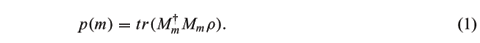The density operator after obtaining the result m is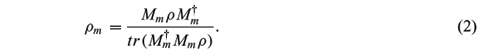The measurement operators satisfy the completeness equation, åmMm = I. The measurement operators are not necessary orthogonal and the number of available outcomes may differ from the dimension N of the Hilbert space. Let us now consider the hermitian, positive operators Em ºMm. It is plain that åmEm = I and that p(m) = tr(Emr). Thus the set of operators Em are sufficient to determine the probabilities p(m) of the different measurement outcomes. The complete set {Em} is known as a POVM (Nielsen and Chuang 2000). Given an arbitrary set of hermitian positive operators {Em} such that åmEm = I, one can show that there exists at least one set of measurement operators Mm defining a measurement described by the POVM {Em}. For instance, we have Mm =|jñáj|, where the |jñ's and lj's, j = 1,2,...N, are the eigenvectors and eigenvalues of Em, respectively. Another possible set of measurement operators Mm is given by Mm = Um, where the Um are arbitrary unitary operators.

A QUANTIZATION SCHEME FOR THE MONTY HALL PROBLEM

The well-known classical Monty Hall problem was originally set in the context of a game show (von Baeyer 2003, D'Ariano et al. 2002).

1. Before the show starts a prize is hidden behind one of three closed boxes. The show master knows where the prize is but, of course, the candidate does not.

2. The candidate is asked to choose one of the three boxes, which is, however, not opened at this stage.

3. The show master opens another box, and shows that it is empty. (He can do this, because he knows where the prize is.)

4. The candidate can now open one of the remaining boxes to either collect her prize or lose.

The question is now, whether the candidate should stick to her original choice or change to the other remaining box. Classically, the optimum strategy is to alter her choice of box, thereby doubling her chance of winning the prize. Even if there is a general consensus that this conclusion is correct, the probabilistic reasoning leading to it is rather subtle, and has been the focus of heated controversy (von Baeyer 2003, Hoffman 1999). Like other probabilistic puzzles of this sort it can be "quantized", i.e., its key elements can be translated into a quantum mechanical language. Usually there are different ways of performing these quantizations, depending on what features of the problem are seen as essential, and also on how are the relevant information gathering processes formalized. The MHP is no exception and quantization schemes have already been proposed [see (Li et al. 2001, D'Ariano et al. 2002, Flitney and Abbott 2002)].

Our present purpose is to advance a different quantum version of the MHP based upon POVM. The main quantum variable will be the location of the prize which is a quantum mechanical particle lying in a three-dimensional Hilbert space. Thus, the particle's Hilbert space is spanned by the orthonormal basis |0ñ, |1ñ and |2ñ, the state |iñ corresponding to the particle in the i-box. Person A then selects a superposition of these states as the initial state of the particle and person B selects a particular box, say |0ñ. This initial choice (|0ñ) stays fixed throughout the problem. Since now either box |1ñ or |2ñ can be opened (opening a box corresponds to a POVM measurement), there will be two possible measurement outcomes: employment of the POVM formalism entails that there will be two operators E1 and E2 that are, precisely, the POVM elements associated with measurement outcomes 1 and 2. In the present quantum mechanical scenario, person A performs the POVM measurement (E1, E2) in order to decide which box to open. If she gets the outcome E1, she opens box |2ñ and if she gets outcome E2, she opens box |1ñ. From the properties of the POVM elements and from the fact that E1 and E2 form a complete set, it follows that (a) E1 + E2 = I, (b) E1 and E2 are positive, (c) E1 and E2 are Hermitian. Also, since the problem is symmetric with respect to 1 and 2, it means that (d) E1 and E2 are symmetric in 1 and 2. The problem requires that the probability that box |1ñ is opened is zero when the particle is in state |1ñ and similarly for box |2ñ. From that condition it follows that when the particle is in box |1ñ, box |2ñ must be opened, that is, the probability of opening |2ñ must be one. Another condition is that, when the particle is in box |0ñ, the probability of either box |1ñ or |2ñ being opened is the same, as neither box is preferred. Therefore, from the relation Em ºMm and equation (1), one obtains á1|E2|1ñ = á2|E1|2ñ = 0, á1|E1|1ñ = á2|E2|2ñ = 1, and á0|E1|0ñ = á0|E2|0ñ =. These constraints for E1 and E2 imply, after some algebra, that their associated matrices arewhere c and b are complex numbers. The eigenvalues of E1,2 are given by the third order equation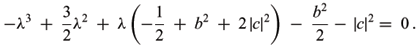The positiveness of E1,2 then requires that b2 + 2 | c |2< 0, implying that c = b = 0. Therefore, the POVM operators Ei =| 0 ñá0 | + | i ñái |, (i = 1, 2) are univocally determined by the basic normalization and symmetry features of the MHP. On the contrary, the measurement operators M1,2 are not univocally determined. The general solutions for the equations E1,2 =M1,2 determining the measurement operators M1,2 compatible with the POVM operators E1,2 are of the form M1 =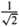| f1ñá0 | + | y1ñá1 | and M2 =| f2ñá0 | + | y2ñá1 |, where | f1ñ, | y1ñ, | f2ñ, and | y2ñ are arbitrary (normalized) states verifying áf1 | y1ñ = 0 and áf2 | y2ñ = 0.

On the basis of the above results we see that various quantum versions of the MHP are possible (depending on the choice of the initial state rin and the measurement operators M1,2) leading to different post-measurement outcomes, with different associated probabilities. Notice that the classical version of the MHP also admits several variants, corresponding to different initial probability distributions for the three boxes, the usual case being that of equiprobability. An initial equiprobable state in the quantum case would be described by a density matrix of the formand I stands for the three-dimensional identity matrix. The quantum MHP proceeds as follows. Person A chooses an initial state rin and then performs the quantum measurement {M1, M2} (assuming that the box selected by B was 0). The state raft after the measurement is (depending on the measurement outcome) given by equation (2). The probabilities of finding the particle in one of the states {| 0ñ, | 1ñ, | 2ñ} are ák | raft | kñ, k = 0, 1, 2. Adopting the initial "equiprobable" state re and M1,2 =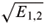it can be shown, after some algebra, that these final probabilities lead to the same conclusion (i.e., person B must change her initial choice in order to double her chances of success) as on the classical way if reasoning. It is interesting that this result does not depend on the value of the parameter l.

It is worth to stress the main features in which our present quantum version of the Monty Hall problem differs from the other quantum versions already discussed in the literature. First of all, ours is a direct quantization of the MHP without recourse to ancillary particles (as opposed, for instance, to the quantization advanced in (Li et al. 2001)). In this respect, the present approach has some similarities with the quantization scheme proposed in (Flitney and Abbott 2002).

However, our quantum version of the Monty Hall game also exhibits important differences from the one discussed in (Flitney and Abbott 2002). In this last case, the quantum game is modelled on the basis of a composite quantum system consisting of three qutrits: one associated with the location of the prize, a second one corresponding the candidate's choice and a third one associated with the master's choice regarding which box is to be open (Flitney and Abbott 2002). Our approach can be regarded as more economical. The state of the system is here described by just one qutrit representing the location of the prize, and the combined choices of the candidate and the master determine the structure of the generalized quantum measurement M1, M2 (and the associated POVM E1, E2).

CONCLUSIONS

A "minimalist" quantum version of the MHP, based upon the POVM formalism, has been advanced. The corresponding POVM elements E1,2 were seen to be completely determined by the basic normalization and symmetry properties of the MHP scenario.

Starting from the an initial "equiprobable" state (with the natural choice M1,2 =for the measurement operators) leads to identical conclusions, concerning the MHP puzzle, as in the classical probabilistic reasoning. It is remarkable that the argument leading to these conclusions seems to be more straightforward in the quantum than in the classical setting. It is perhaps not too farfetched to assert that the correct answer to the MHP puzzle is "embodied" in the very formalism of quantum mechanic's measurement theory.

ACKNOWLEDGMENTS

This work was partially supported by the MEC grant FIS2005-02796 (Spain), and by CONICET (Argentine Agency).

Manuscript received on December 21, 2005; accepted for publication on April 17, 2006; contributed by ANGEL PLASTINO*

* Member Academia Brasileira de Ciências

Correspondence to: A. Plastino

E-mail: plastino@fisica.unlp.edu.ar

• D'ARIANO GM, GILL RD, KEYL M, KUEMMERER B, MAASSEN H AND WERNER RF. 2002. The quantum Monty Hall problem. Quant Inf Comput 2: 355-366.
• FLITNEY AP AND ABBOTT D. 2002. Quantum Version of the Monty Hall Problem. Phys Rev A 65: 062318.
• FRIEDEN BR. 2004. Science from Fisher Information. Cambridge: Cambridge University Press.
• GILLMAN L. 1992. The Car and the Goat. Amer Math Month 99: 3-7.
• GLAZER M AND WORK J. 2001. Statistical Mechanics, Oxford: Oxford University Press
• HOFFMAN P. 1999. The Man Who Loved Only Numbers. New York: Hyperion.
• LEFF HS AND REX AF (Eds). 2003. Maxwell's Demon II: entropy, Classical and Quantum Information, Computing, Bristol and Philadelphia: Institute of Physics Publishing.
• LI C-F, ZHANG Y-S, HUANG Y-F AND GUO G-C. 2001. Quantum Strategies of Quantum Measurement. Phys Lett A 280: 257-260.
• MEYER DA. 1999. Quantum Strategies. Phys Rev Lett 82: 1052-1055.
• NIELSEN MA AND CHUANG IL. 2000. Quantum Computation and Quantum Information, Cambridge: Cambridge University Press, p. 84-96
• PLASTINO AR AND DAFFERTSHOFER A. 2004. Liouville dynamic and the conservation of classical information. Phys Rev Lett 93: 138701-138704.
• TSALLIS C, DEUTSCHER G AND MAYNAR R. 1996. On probabilities and information - The envelope game. REBRAPE/. Braz J Prob Stat 10: 103-109.
• VON BAEYER HC. 2003. Information: The New Language of Science. London: Weidenfeld and Nicolson.

Publication Dates

• Publication in this collection
18 Aug 2006
• Date of issue
Sept 2006

• Accepted
17 Apr 2006